Successfully reported this slideshow.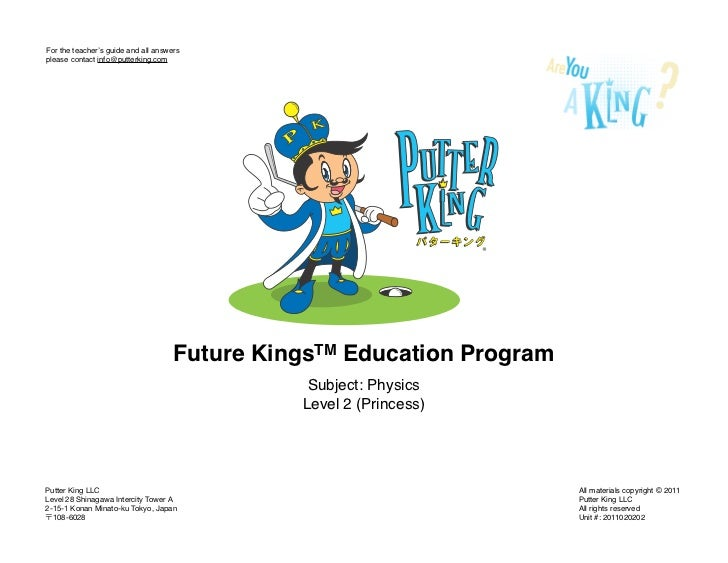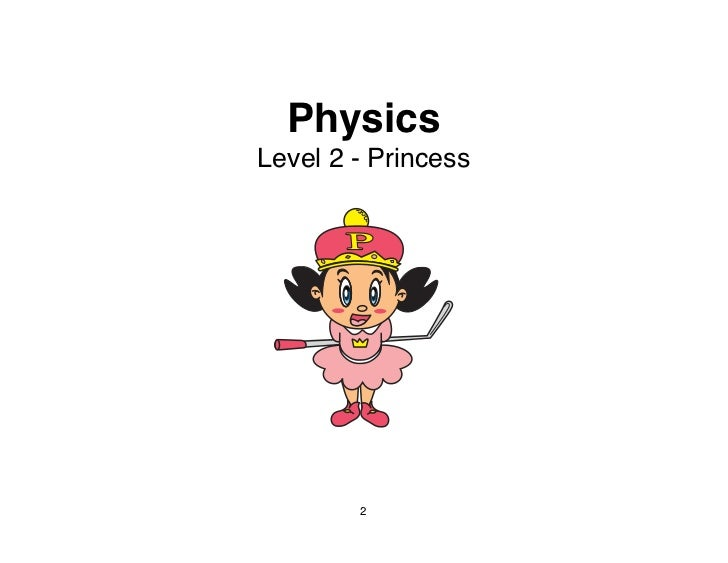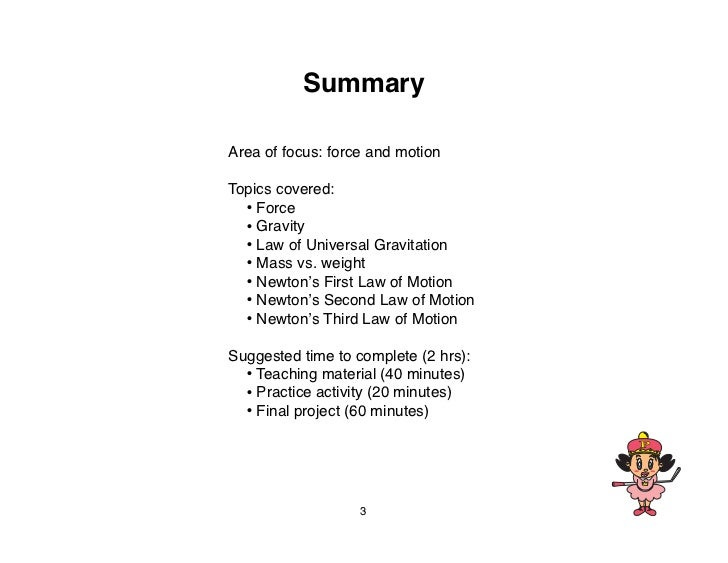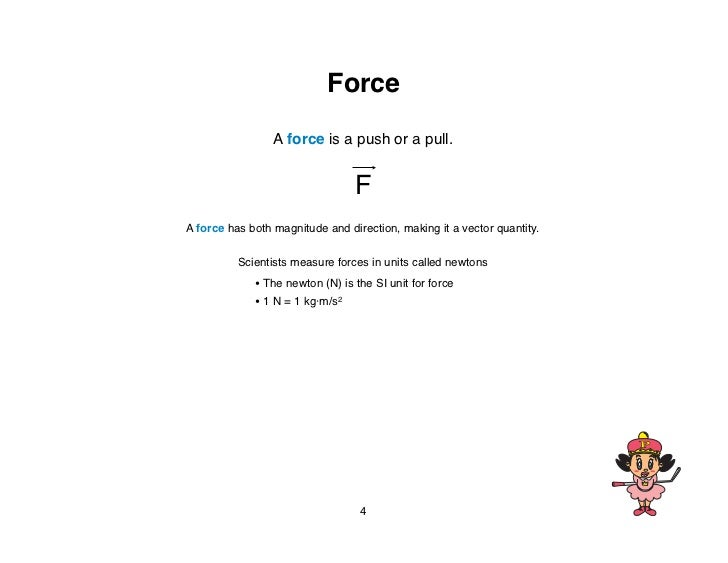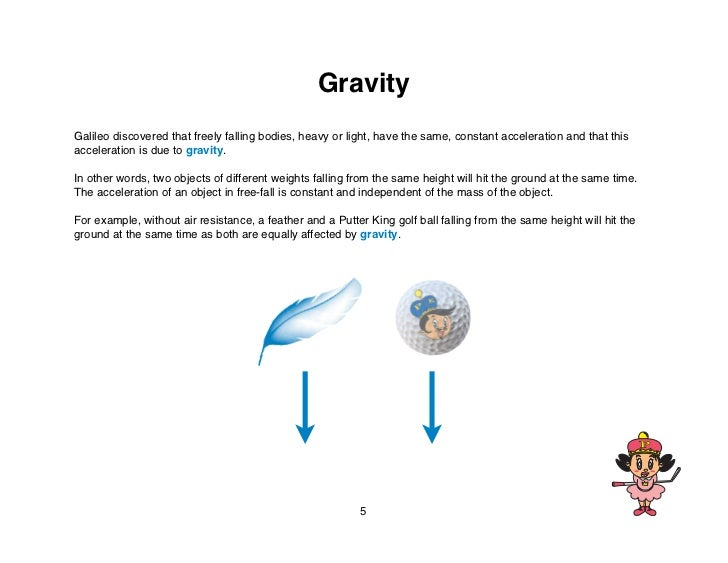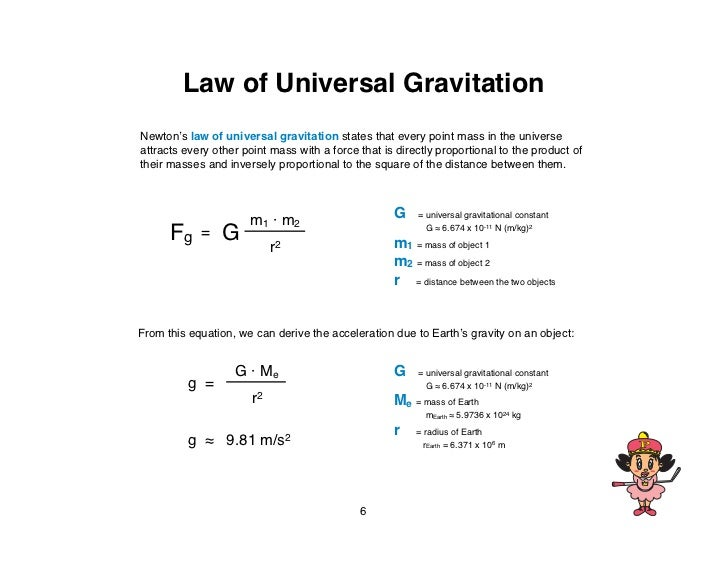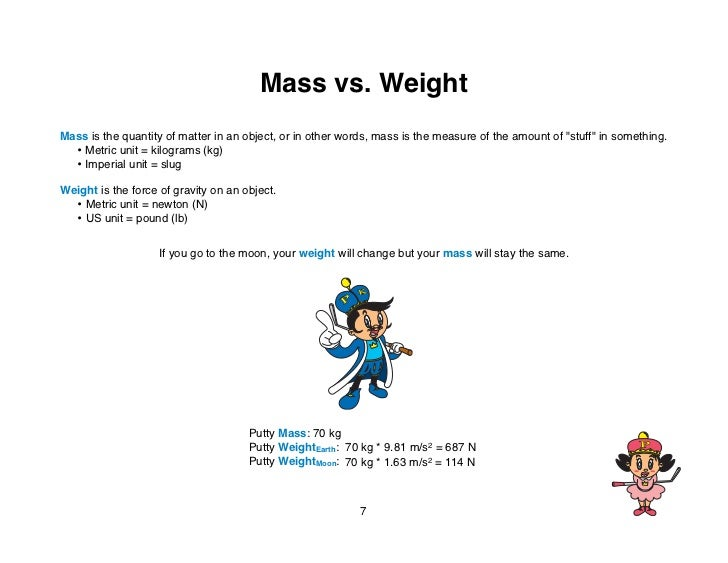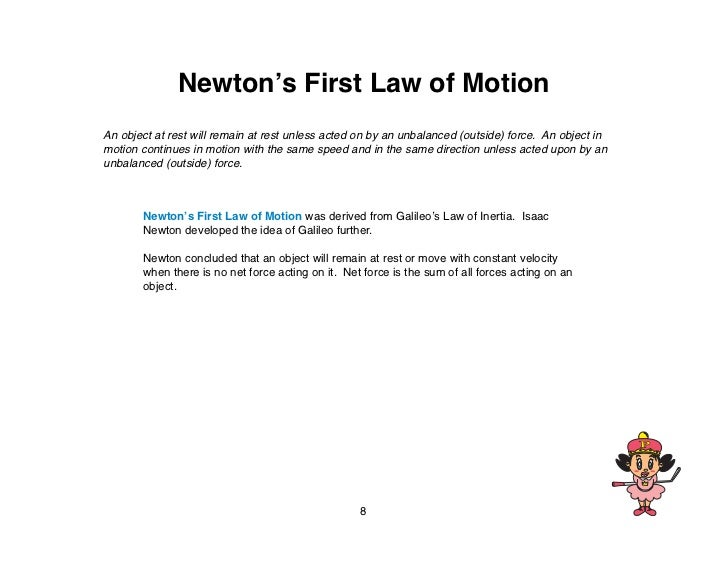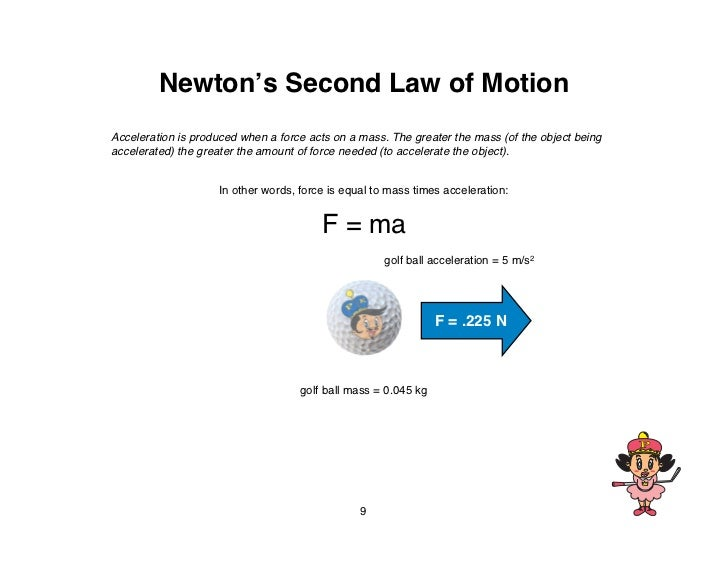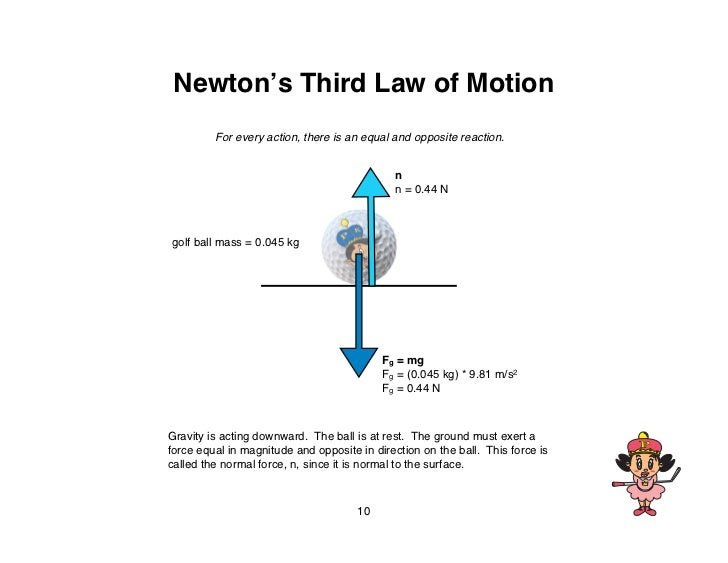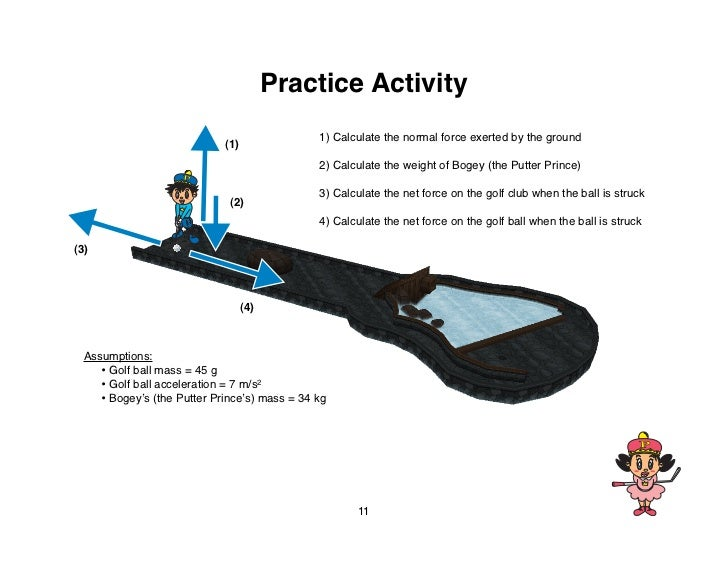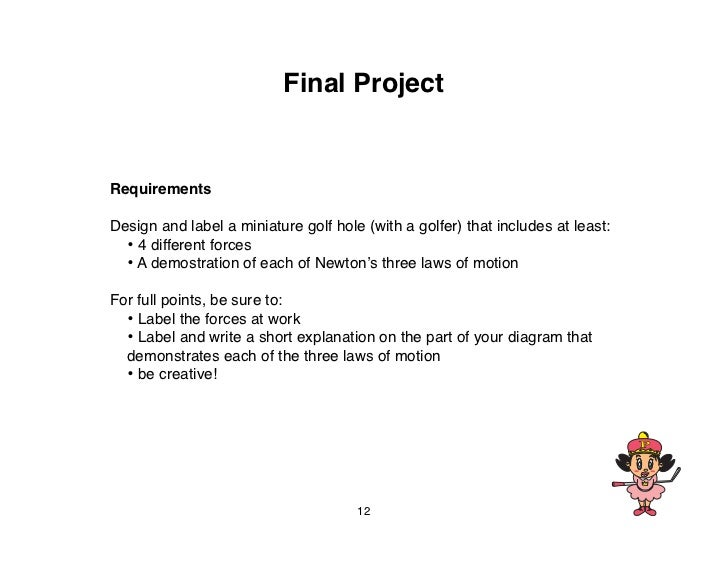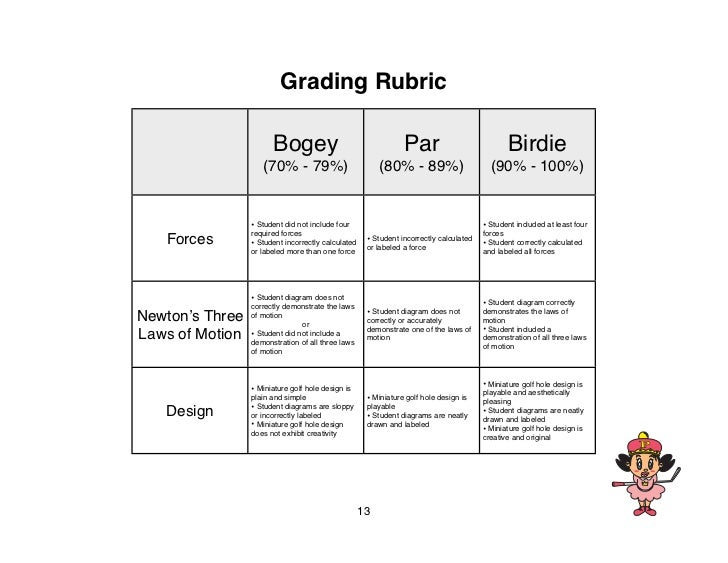Upcoming SlideShare
×
• Full Name
Comment goes here.

Are you sure you want to Yes No• Be the first to comment

### Putter King Education - Physics (Level 2)

2. 2. PhysicsLevel 2 - Princess 2
3. 3. SummaryArea of focus: force and motionTopics covered: • Force • Gravity • Law of Universal Gravitation • Mass vs. weight • Newtonʼs First Law of Motion • Newtonʼs Second Law of Motion • Newtonʼs Third Law of MotionSuggested time to complete (2 hrs): • Teaching material (40 minutes) • Practice activity (20 minutes) • Final project (60 minutes) 3
4. 4. Force A force is a push or a pull. FA force has both magnitude and direction, making it a vector quantity. Scientists measure forces in units called newtons • The newton (N) is the SI unit for force • 1 N = 1 kg·m/s2 4
5. 5. GravityGalileo discovered that freely falling bodies, heavy or light, have the same, constant acceleration and that thisacceleration is due to gravity.In other words, two objects of different weights falling from the same height will hit the ground at the same time.The acceleration of an object in free-fall is constant and independent of the mass of the object.For example, without air resistance, a feather and a Putter King golf ball falling from the same height will hit theground at the same time as both are equally affected by gravity. 5
6. 6. Law of Universal GravitationNewtonʼs law of universal gravitation states that every point mass in the universeattracts every other point mass with a force that is directly proportional to the product oftheir masses and inversely proportional to the square of the distance between them. m1 · m2 G = universal gravitational constant Fg = G G ≈ 6.674 x 10-11 N (m/kg)2 m1 = mass of object 1 r2 m2 = mass of object 2 r = distance between the two objectsFrom this equation, we can derive the acceleration due to Earthʼs gravity on an object: G · Me G = universal gravitational constant g = G ≈ 6.674 x 10-11 N (m/kg)2 r2 Me = mass of Earth mEarth ≈ 5.9736 x 1024 kg r = radius of Earth g ≈ 9.81 m/s2 rEarth = 6.371 x 106 m 6
7. 7. Mass vs. WeightMass is the quantity of matter in an object, or in other words, mass is the measure of the amount of "stuff" in something. • Metric unit = kilograms (kg) • Imperial unit = slugWeight is the force of gravity on an object. • Metric unit = newton (N) • US unit = pound (lb) If you go to the moon, your weight will change but your mass will stay the same. Putty Mass: 70 kg Putty WeightEarth: 70 kg * 9.81 m/s2 = 687 N Putty WeightMoon: 70 kg * 1.63 m/s2 = 114 N 7
8. 8. Newtonʼs First Law of MotionAn object at rest will remain at rest unless acted on by an unbalanced (outside) force. An object inmotion continues in motion with the same speed and in the same direction unless acted upon by anunbalanced (outside) force. Newtonʼs First Law of Motion was derived from Galileoʼs Law of Inertia. Isaac Newton developed the idea of Galileo further. Newton concluded that an object will remain at rest or move with constant velocity when there is no net force acting on it. Net force is the sum of all forces acting on an object. 8
9. 9. Newtonʼs Second Law of MotionAcceleration is produced when a force acts on a mass. The greater the mass (of the object beingaccelerated) the greater the amount of force needed (to accelerate the object). In other words, force is equal to mass times acceleration: F = ma golf ball acceleration = 5 m/s2 F = .225 N golf ball mass = 0.045 kg 9
10. 10. Newtonʼs Third Law of Motion For every action, there is an equal and opposite reaction. n n = 0.44 Ngolf ball mass = 0.045 kg Fg = mg Fg = (0.045 kg) * 9.81 m/s2 Fg = 0.44 NGravity is acting downward.  The ball is at rest.  The ground must exert aforce equal in magnitude and opposite in direction on the ball.  This force iscalled the normal force, n, since it is normal to the surface. 10
11. 11. Practice Activity 1) Calculate the normal force exerted by the ground (1) 2) Calculate the weight of Bogey (the Putter Prince) 3) Calculate the net force on the golf club when the ball is struck (2) 4) Calculate the net force on the golf ball when the ball is struck(3) (4) Assumptions: • Golf ball mass = 45 g • Golf ball acceleration = 7 m/s2 • Bogeyʼs (the Putter Princeʼs) mass = 34 kg 11
12. 12. Final ProjectRequirementsDesign and label a miniature golf hole (with a golfer) that includes at least: • 4 different forces • A demostration of each of Newtonʼs three laws of motionFor full points, be sure to: • Label the forces at work • Label and write a short explanation on the part of your diagram that demonstrates each of the three laws of motion • be creative! 12
13. 13. Grading Rubric Bogey Par Birdie (70% - 79%) (80% - 89%) (90% - 100%) • Student did not include four • Student included at least four required forces forces Forces • Student incorrectly calculated • Student incorrectly calculated or labeled a force • Student correctly calculated or labeled more than one force and labeled all forces • Student diagram does not • Student diagram correctly correctly demonstrate the laws • Student diagram does not demonstrates the laws ofNewtonʼs Three of motion or correctly or accurately motion demonstrate one of the laws of • Student included aLaws of Motion • Student did not include a demonstration of all three laws motion demonstration of all three laws of motion of motion • Miniature golf hole design is • Miniature golf hole design is playable and aesthetically plain and simple • Miniature golf hole design is pleasing • Student diagrams are sloppy playable Design or incorrectly labeled • Student diagrams are neatly • Student diagrams are neatly drawn and labeled • Miniature golf hole design drawn and labeled • Miniature golf hole design is does not exhibit creativity creative and original 13

### Be the first to comment

•#### ssusere480c0

Aug. 6, 2015
•#### PhilipSolomon4

Jun. 19, 2017
•Nov. 1, 2017

Total views

3,035

On Slideshare

0

From embeds

0

Number of embeds

1,424

55

Shares

0